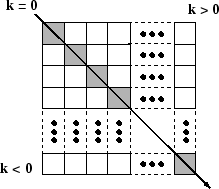# diag

## 语法

``D = diag(v)``
``D = diag(v,k)``
``x = diag(A)``
``x = diag(A,k)``

## 说明

``D = diag(v)` 返回包含主对角线上向量 `v` 的元素的对角矩阵。`

``D = diag(v,k)` 将向量 `v` 的元素放置在第 `k` 条对角线上。`k=0` 表示主对角线，`k>0` 位于主对角线上方，`k<0` 位于主对角线下方。`

``x = diag(A)` 返回 `A` 的主对角线元素的列向量。`

``x = diag(A,k)` 返回 `A` 的第 `k` 条对角线上元素的列向量。`

## 示例

`v = [2 1 -1 -2 -5];`

`D = diag(v)`
```D = 5×5 2 0 0 0 0 0 1 0 0 0 0 0 -1 0 0 0 0 0 -2 0 0 0 0 0 -5 ```

`D1 = diag(v,1)`
```D1 = 6×6 0 2 0 0 0 0 0 0 1 0 0 0 0 0 0 -1 0 0 0 0 0 0 -2 0 0 0 0 0 0 -5 0 0 0 0 0 0 ```

`A = randi(10,6)`
```A = 6×6 9 3 10 8 7 8 10 6 5 10 8 1 2 10 9 7 8 3 10 10 2 1 4 1 7 2 5 9 7 1 1 10 10 10 2 9 ```
`x = diag(A)`
```x = 6×1 9 6 9 1 7 9 ```

`x1 = diag(A,-1)`
```x1 = 5×1 10 10 2 9 2 ```

`A1 = diag(diag(A))`
```A1 = 6×6 9 0 0 0 0 0 0 6 0 0 0 0 0 0 9 0 0 0 0 0 0 1 0 0 0 0 0 0 7 0 0 0 0 0 0 9 ```

## 输入参数

`diag([])` 返回空矩阵 `[]`

`diag([])` 返回空矩阵 `[]`